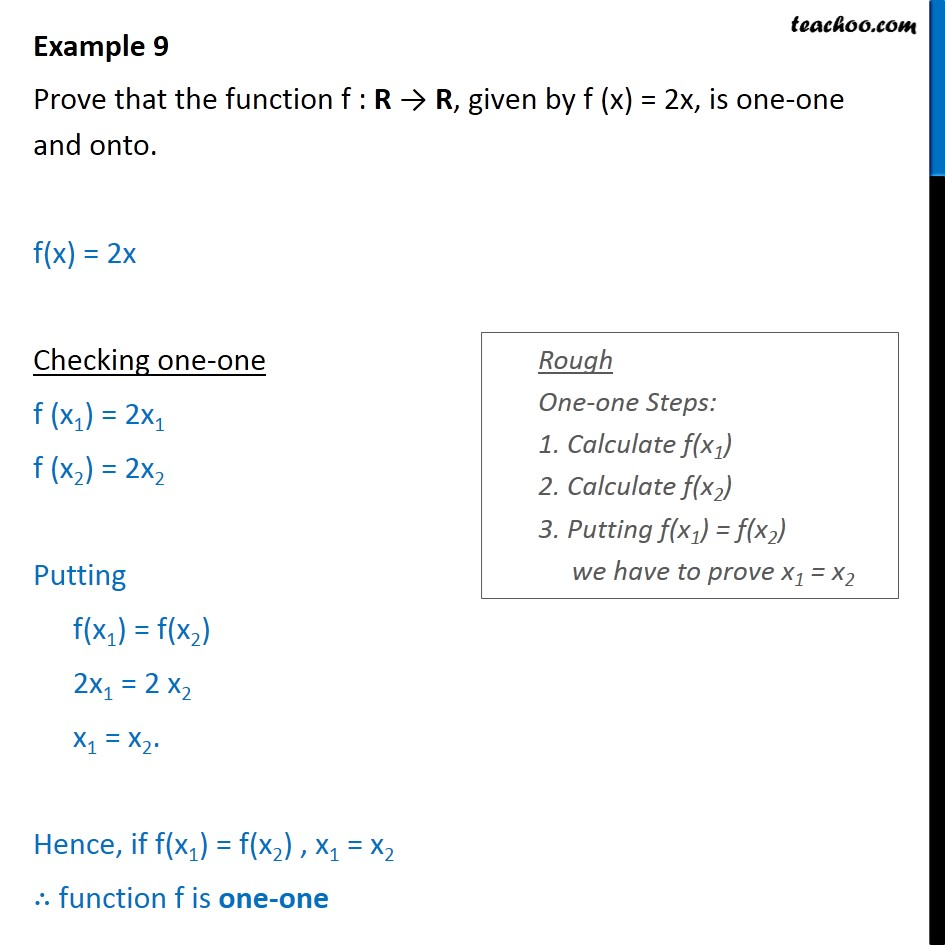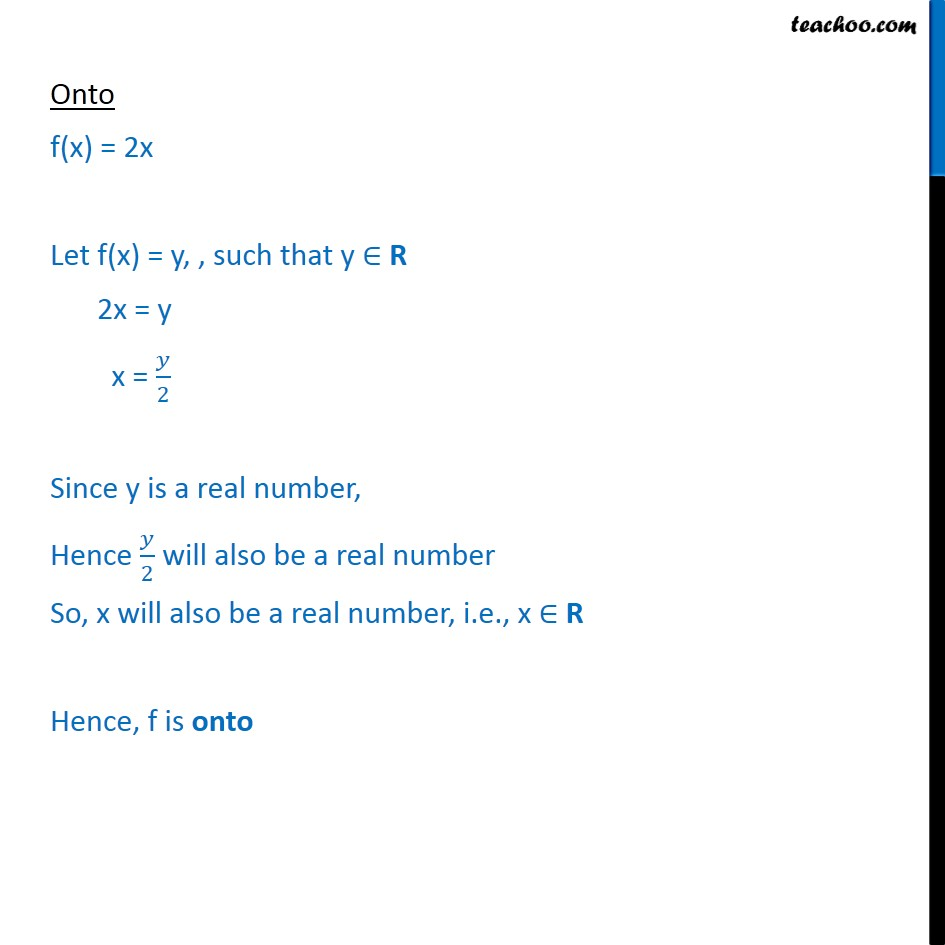Examples

Chapter 1 Class 12 Relation and Functions (Term 1)
Serial order wiseNow learn Economics at Teachoo for Class 12

### Transcript

Example 9 Prove that the function f : R → R, given by f (x) = 2x, is one-one and onto. f(x) = 2x Checking one-one f (x1) = 2x1 f (x2) = 2x2 Putting f(x1) = f(x2) 2x1 = 2 x2 x1 = x2. Hence, if f(x1) = f(x2) , x1 = x2 ∴ function f is one-one Onto f(x) = 2x Let f(x) = y, , such that y ∈ R 2x = y x = 𝑦﷮2﷯ Since y is a real number, Hence 𝑦﷮2﷯ will also be a real number So, x will also be a real number, i.e., x ∈ R Hence, f is onto